Courses

# Test: Structural of Metals & Alloys - 3

## 20 Questions MCQ Test Engineering Materials | Test: Structural of Metals & Alloys - 3

Description
This mock test of Test: Structural of Metals & Alloys - 3 for Mechanical Engineering helps you for every Mechanical Engineering entrance exam. This contains 20 Multiple Choice Questions for Mechanical Engineering Test: Structural of Metals & Alloys - 3 (mcq) to study with solutions a complete question bank. The solved questions answers in this Test: Structural of Metals & Alloys - 3 quiz give you a good mix of easy questions and tough questions. Mechanical Engineering students definitely take this Test: Structural of Metals & Alloys - 3 exercise for a better result in the exam. You can find other Test: Structural of Metals & Alloys - 3 extra questions, long questions & short questions for Mechanical Engineering on EduRev as well by searching above.
QUESTION: 1

Solution:
QUESTION: 2

### Atomic packing factor in case of Copper Crystal is

Solution:

Since Copper .is having FCC structure APF = 0.74

QUESTION: 3

### The effective number of lattice points in the unit celi of simple cubic, body centered cubic and face centered cubic space lattices respectively are

Solution:

For simple cubic effective number of lattice point = 1
For B.C.C. effective number of lattice point = 2
For F.C.C. effective number of lattice point = 4

QUESTION: 4

Which of the following elements is not a metalloid?

Solution:
QUESTION: 5

Which of the following material is not a ferromagnetic material?

Solution:
QUESTION: 6

Which one of the following pairs of axis lengths (a, b, c) and inter axial angles (a, β, γ) represents the tetragonal crystal system?

Solution: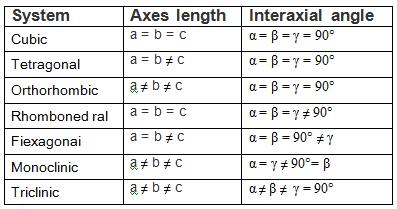QUESTION: 7

The defect which takes place due to imperfect packing of atoms during crystallization is known as

Solution:
QUESTION: 8

What is the movement of block of atoms along certain crystallographic phase and directions termed as

Solution:

Slip is defined as a irreversible shear displacement of one part of a crystal relative to another in a definite crystallographic direction and an a specific crystallographic plane.

QUESTION: 9

A screw dislocation
1. Lies parallel to its Burger’s vector
2. Lies perpendicular to its Burger’s vector
3. Moves in a perpendicular direction to the Burger’s vector
4. Moves in an inclined direction to the Burger’s vector
Q. Select the correct answer using the codes given below:

Solution:

Screw dislocation lies parallel to Burger vector and moves in perpendicular direction to Burger vector.
Edge dislocation - Burger vector in normal to dislocation line and movement will be parallel to Burger vector.

QUESTION: 10

The set of miller indices of the plane shown in the given figure is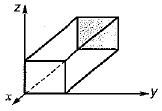Solution:

Intercept on y and z-axis is ∞. So taking reciprocal it will be 0, 0 on both y and z-axis. Intercept on x-axis is on the negative side of x-axis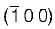.

QUESTION: 11

Match List-I (Element) with List-ll (Crystal structure) and select the correct answer using the codes given below the lists:
List-I
A. Alpha Iron
B. Copper
C. Zinc
D. Glass
List-ll
1. Hexagonal closed packed
2. Body-centered cubic
3. Amorphous
4. Face-centered cubic
Codes:
A B C D
(a) 2 3 1 4
(b) 1 4 2 3
(c) 2 4 1 3
(d) 1 3 2 4

Solution:
QUESTION: 12

What is the planer density of (100) plane in FCC (face-centred cubic) crystal with unit cell side a equa! to?

Solution:

FCC on (100) plane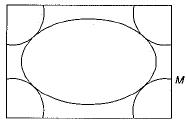No. of atoms = 1/4 x 4 +1 = 2
Area = a x a = a2
Planar density = 2/a2

QUESTION: 13

A unit cell of a crystal is shown in given figure. The miller indices of the direction (arrow) shown in the figure is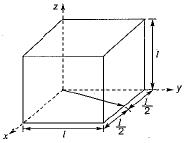Solution:
QUESTION: 14

Match List-I (Miller indices) with List-ll (Denotes) and select the correct answer using the codes given below the lists:
List-I
A. (h, k l)
B. [h k l]
C. {h k l}
D. <h k l>
List-ll
1. direction
2. plane
3. family of direction
4. Family of planes
Codes:
A B C D
(a) 1 2 3 4
(b) 1 2 4 3
(c) 2 1 4 3
(d) 2 1 3 4

Solution:
QUESTION: 15

Planar density in SC on (100) plane is

Solution: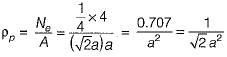QUESTION: 16

Planar density in BCC on (111) plane is

Solution: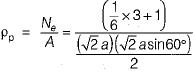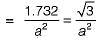QUESTION: 17

The linear density along the direction (110) will be equal to, if lattice constant of copper unit cell is 3.61 Å.

Solution: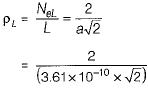= 3.91 x 109 atoms/m
ρ = 3.91 x 106 atoms/mm

QUESTION: 18

Miller indices of a plane will be whose intercepts are a, b/2 and 3c on x, y and z axes respectively in a simple cubic unit cell?

Solution:
QUESTION: 19

Consider the following statements about screw dislocation
1. It forms when crystal displaces angulanly over the remaining parts
2. Burgers vector is parallel to screw dislocation line
3. Screw dislocations are symbolically represented by clockwise & anticiock-wise and reffered to negative & positive screw dislocation respectively
Q. Which of these statement are correct?

Solution:
QUESTION: 20

Ratio of elastic strain energy of an edge dislocation to the screw dislocation is

Solution: